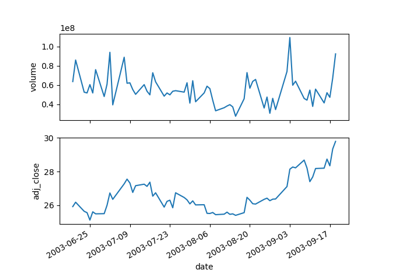# matplotlib.pyplot.plotfile¶

`matplotlib.pyplot.``plotfile`(fname, cols=(0, ), plotfuncs=None, comments='#', skiprows=0, checkrows=5, delimiter=', ', names=None, subplots=True, newfig=True, **kwargs)

Plot the data in a file.

cols is a sequence of column identifiers to plot. An identifier is either an int or a string. If it is an int, it indicates the column number. If it is a string, it indicates the column header. matplotlib will make column headers lower case, replace spaces with underscores, and remove all illegal characters; so `'Adj Close*'` will have name `'adj_close'`.

• If len(cols) == 1, only that column will be plotted on the y axis.
• If len(cols) > 1, the first element will be an identifier for data for the x axis and the remaining elements will be the column indexes for multiple subplots if subplots is True (the default), or for lines in a single subplot if subplots is False.

plotfuncs, if not None, is a dictionary mapping identifier to an `Axes` plotting function as a string. Default is ‘plot’, other choices are ‘semilogy’, ‘fill’, ‘bar’, etc. You must use the same type of identifier in the cols vector as you use in the plotfuncs dictionary, e.g., integer column numbers in both or column names in both. If subplots is False, then including any function such as ‘semilogy’ that changes the axis scaling will set the scaling for all columns.

comments, skiprows, checkrows, delimiter, and names are all passed on to `matplotlib.pylab.csv2rec()` to load the data into a record array.

If newfig is True, the plot always will be made in a new figure; if False, it will be made in the current figure if one exists, else in a new figure.

kwargs are passed on to plotting functions.

Example usage:

```# plot the 2nd and 4th column against the 1st in two subplots
plotfile(fname, (0,1,3))

# plot using column names; specify an alternate plot type for volume
## Examples using `matplotlib.pyplot.plotfile`¶